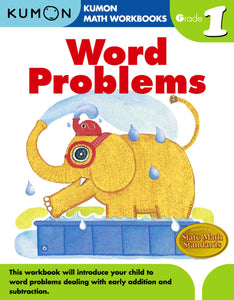# Kumon Word Problems

Regular price ₱290.00 Sale price ₱2,300.00 Unit price per

Grade 1- Word Problems' series designed for grades 1-6. This workbook will introduce students to word problems dealing with early addition and subtraction. Exercises use visual illustrations before moving on to problems with illustrations and questions that are only written. Designed for students to self-score their work, pages provide the number of points to assign per problem, and there's room at the top to write in how many problems they got right alongside their name and the date.

Grade 2- This workbook guides your child through the basics of answering word problems with large numbers. Length and weight word problems give children real world addition and subtraction applications. Through our gradual progression of activities, your child will advance to tables and graphs with ease. Addition and subtraction Reading graphs Drawing graphs and counting given concrete

Grade 3- This workbook helps your child build a strong foundation in word problems with multiplication and division. Children will progress gradually through our activities, to confidently choose which operations to use to solve word problems. Then, by mastering graphs and tables, your child will advance to more difficult word problems with ease. -Addition, subtraction, multiplication, and

Grade 4- This workbook guides children through more sophisticated word problems that include fractions and decimals. Also, mixed calculation sections incorporate multiple-digit multiplication and division, as well as line graphs and pictographs. Multiplication and division of whole numbers Fractions and decimals Estimation (round numbers, addition and subtraction) Reading and writing line graphs and pictographs Determining number outcomes

Grade 5- In Grade 5 Word Problems, your child will complete word problems with decimals, percentages, ratios, and graphs. By reviewing important skills such as rounding numbers and identifying odd and even numbers, this workbook builds confidence as children continue to improve their word problem abilities. Fractions and decimals Addition and subtraction of measurements Determining percentage

Grade 6- This workbook familiarizes children with complex arithmetic concepts. Your child will solve problems dealing with speed, distance, and time. By mastering probability and averages, your child can advance to more difficult word problems with ease. Fractions Solving percent problems including percent, rate, and base Solving problems including speed, distance, time Determining probability Determining an average